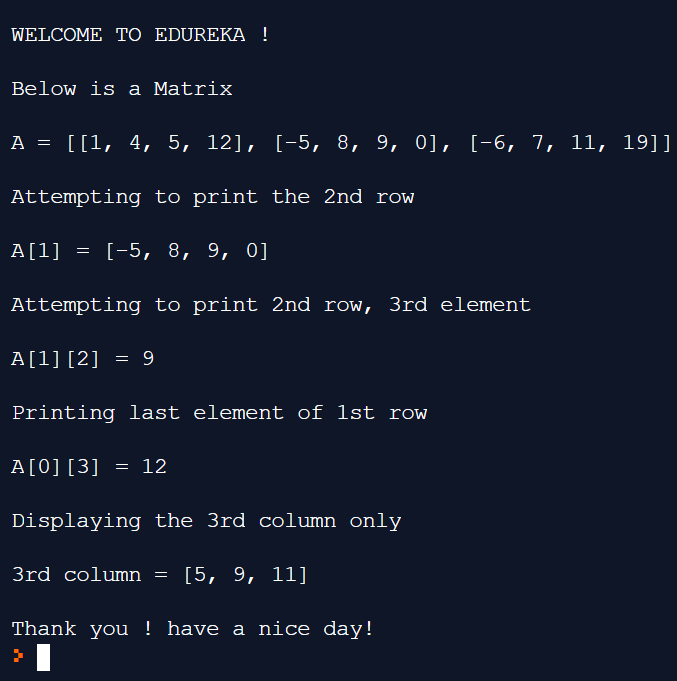# Everything You Need To Know About Matrix In PythonThis article will introduce you Matrix in Python with every operation that concerns the topic with a programmatic demonstration. Following pointers will be covered in this article,

Let us get started then,

Matrix In Python

Matrix is nothing but a rectangular array of numbers or any other form of data. The basic concept of a matrix should be clear before operating on matrices within the boundaries of python programming language. The horizontal arrangement of data are rows and the vertical arrangement are columns. The size of any matrices or in other words, the number of elements inside a matrix is (R) X (C) where R is rows and C, columns. Python does not have a built in type for matrices, so we consider two or more lists together as a matrix.

Now, let us have a look at viewing elements of a matrix and its functionality. Consider the below illustrated python code.

```print("nWELCOME TO EDUREKA !n")
print("Below is a Matrixn")
A= [[1,4,5,12],
[-5,8,9,0]
[-6,7,11,19]]
print("A=", A)
print("nAttempting to print the 2nd rown")
print("A =",  A)
print("nAttempting to print the 2nd row, 3rd elementn")
print("A=", A)
print("nPriting last element of the 1st rown")
print("A =", A)
column = [];
for row in A:
column.append(row)
print("n Displaying the 3rd column onlyn")
print("3rd column=", column)
print("n Thank you ! Have a nice day!")```

OutputMoving on with this article

NumPy Package For Matrices In Python

Numpy is a python library, which allows for scientific computing. Numpy can help users work on multidimensional arrays.

```/Addition of Matrices
print("nWELCOME TO EDUREKA!n")
import numpy as np
A= np.array([[24,41],[35,-9]])
B= np.array([[19,-36],[37,68]])
C=A+B
print("Summing a matrix using Numpy is simplen")
print(C)
print("nThank You!")```

Output

/Multiplication Of Matrices

The product of two matrices is found using Numpy libraries as illustrated below.

```//
Import numpy as np
A= np.array([[7,1,3],[6,-2,0]])
B= np.array([[2,3],[9,5],[4,-2]])
C=A.dot(B)
print("nThe product of two matrices is n")
print(C)
print("nThank you !n")```

OutputTranspose Of A Matrix

Transpose refers to a new matrix formed whose rows are now the columns and whose columns are now the rows of the initial matrix.

```//
Import numpy as np
A=np.array([[1,1],[2,1],[3,-3]])
print(“n This is your original matrixn”)
print(A)
print(A.transpose())
print(“nThank You”)```

OutputThis brings us to the end of this article.

To get in-depth knowledge on Python along with its various applications, you can enroll here for live online training with 24/7 support and lifetime access.

Got a question for us? Mention them in the comments section of  article and we will get back to you.

Upcoming Batches For Python Certification Training Course
Course NameDate
Python Certification Training Course

Class Starts on 30th September,2023

30th September

SAT&SUN (Weekend Batch)
View Details
Python Certification Training Course

Class Starts on 21st October,2023

21st October

SAT&SUN (Weekend Batch)
View DetailsREGISTER FOR FREE WEBINARThank you for registering Join Edureka Meetup community for 100+ Free Webinars each month